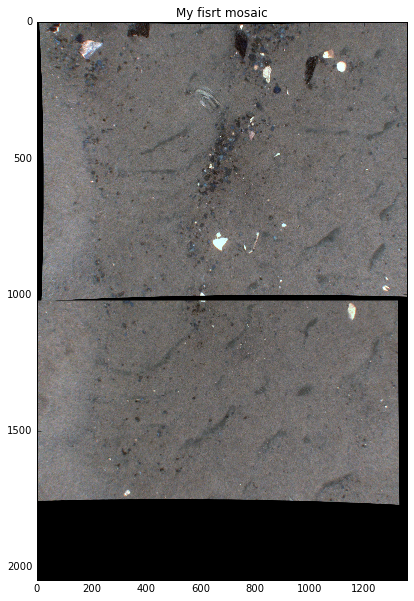In :
%matplotlib inline
from matplotlib import pyplot as plt

In :
import imutils
import cv2
import numpy as np

In :
cv2.__version__

Out:
'3.1.0'
In :
def detectAndDescribe(image):
# convert the image to grayscale
gray = cv2.cvtColor(image, cv2.COLOR_BGR2GRAY)
descriptor = cv2.xfeatures2d.SIFT_create()
(kps, features) = descriptor.detectAndCompute(image, None)
# convert the keypoints from KeyPoint objects to NumPy
# arrays
kps = np.float32([kp.pt for kp in kps])
# return a tuple of keypoints and features
return (kps, features)

In :
def matchKeypoints(kpsA, kpsB, featuresA, featuresB, ratio, reprojThresh):
# compute the raw matches and initialize the list of actual
# matches
matcher = cv2.DescriptorMatcher_create("BruteForce")
rawMatches = matcher.knnMatch(featuresA, featuresB, 2)
matches = []

# loop over the raw matches
for m in rawMatches:
# ensure the distance is within a certain ratio of each
# other (i.e. Lowe's ratio test)
if len(m) == 2 and m.distance < m.distance * ratio:
matches.append((m.trainIdx, m.queryIdx))

# computing a homography requires at least 4 matches
if len(matches) > 4:
# construct the two sets of points
ptsA = np.float32([kpsA[i] for (_, i) in matches])
ptsB = np.float32([kpsB[i] for (i, _) in matches])

# compute the homography between the two sets of points
(H, status) = cv2.findHomography(ptsA, ptsB, cv2.RANSAC,
reprojThresh)

# return the matches along with the homograpy matrix
# and status of each matched point
return (matches, H, status)

# otherwise, no homograpy could be computed
return None

In :
# load the two images
imageA = cv2.imread('1orig.png')
imageB = cv2.imread('2orig.png')


TODO:

Habcam images are "along-track" to adapt to the existing code i'm rotating the images here this is bad because is an extra step in the workflow
In :
#imageB = np.rot90(imageB_v, k=3)
#imageA = np.rot90(imageA_v, k=3)

In :
(kpsA, featuresA) = detectAndDescribe(imageA)
(kpsB, featuresB) = detectAndDescribe(imageB)

In :
# match features between the two images
ratio=0.75
reprojThresh=4.0

M = matchKeypoints(kpsA, kpsB, featuresA, featuresB, ratio, reprojThresh)


TODO:

I need to study the parameters ratio and reprojThresh
In :
(matches, H, status) = M
result = cv2.warpPerspective(imageA, H, (imageA.shape, imageA.shape + imageB.shape))
result[0:imageB.shape, 0:imageB.shape] = imageB

In :
plt.figure(figsize=(10,10))
plt.imshow(result)
plt.title('My fisrt mosaic');In :
plt.figure(figsize=(10,10))
plt.imshow(imageA)
plt.title('First image');